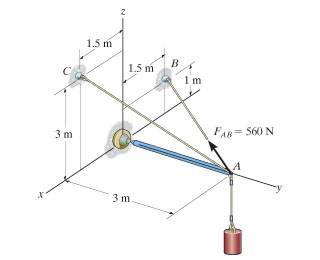# Vector Projection Problem

• Lancelot59

#### Lancelot59I need to find the components of the force along AB along AC.

So I got unit vectors for each like so:

$$\vec{AB}=<-1.5,-3,1>$$
$$\vec{AC}=<-1.5,-3,3>$$

Norm AB=sqrt(12.25). Norm AC=sqrt(20.25).

Then after multiplying the unit vector AB by the force I tried using the vector projection formula:

$$proj_{\vec{AB}}{\vec{AC}}=\frac{\vec{AB}\cdot\vec{AC}}{\norm{AB}\norm{AC}} \vec{AC}$$

But my answer was wrong. I don't know what the right answer is.